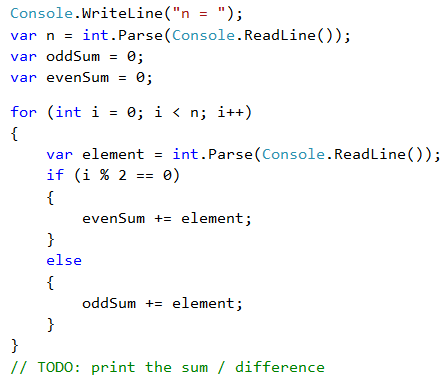# Problem: Even / Odd Sum

Write a program that inputs n integers and checks whether the sum of the numbers on even positions is equal to the sum of the numbers on odd positions. In case the sums are equal, print "Yes" + the sum, otherwise, print "No" + the difference. The difference is calculated by absolute value. The format of the output must be identical to the examples below.

Input Output
4
10
50
60
20
Yes
Sum = 70
4
3
5
1
-2
No
Diff = 1
3
5
8
1
No
Diff = 2

## Video: Even / Odd Sum

Watch this video to learn how to sum the element at even and odd positions and how to calculate their equality or difference: https://youtu.be/79QsS7FI2qg.

## Hints and Guidelines

We input the numbers one by one and calculate the two sums (of the numbers on even positions and the numbers on odd positions). Identically to the previous problem, we calculate the absolute value of the difference and print the result ("Yes" + the sum in case of difference of 0 or "No" + the difference in any other case).## Testing in the Judge System

Test your solution here: https://judge.softuni.org/Contests/Practice/Index/510#7.